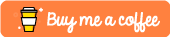#Python Maps Tutorial

This tutorial was built using Python 3.6

Maps in Python are syntactic sugar that allow you to very succinctly iterate through every element of an array and perform a function on it.

Imagine we started off with a list of values numbering from 2-6 like so:

``values = [2,3,4,5,6]``

And we had a function which doubled said values:

``````def double(x):
return x * 2``````

Without the use of maps we would have to do something like so in order to multiply every element:

``````for value in values:
value = double(value)``````

but with maps we can be far more succinct:

``results = list(map(double, values))``

## Complete Example

Below you’ll find a complete exmaple for a simple map which applies the double function to every element in the values array.

``````values = [2,3,4,5,6]

def double(x):
return x * 2

results = list(map(double, values))
print(results)``````

this produces the following output:

``[4, 6, 8, 10, 12, 14, 16]``

# Conclusion

Hopefully you found this tutorial useful! If you did or you require further assistance then please feel free to let me know in the comments section below or by tweeting me @Elliot_F.